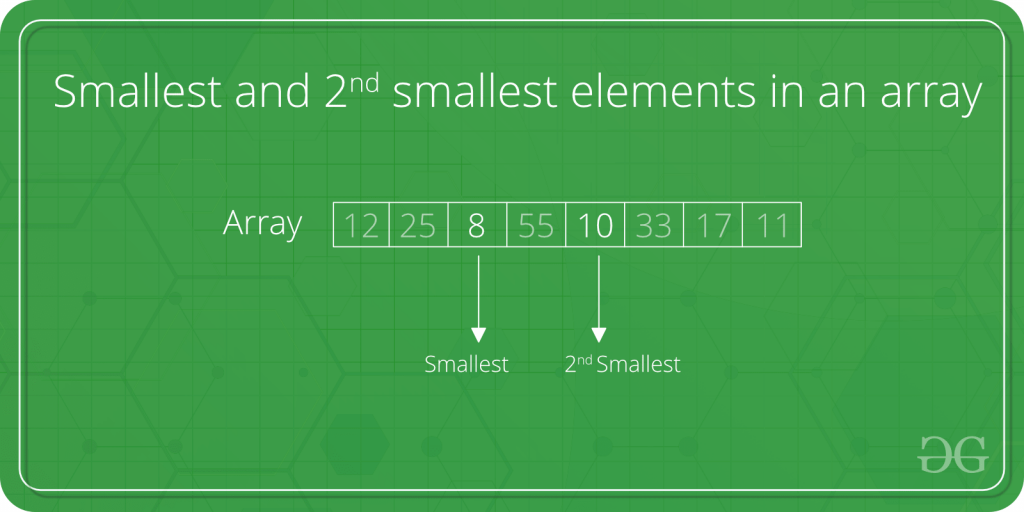Find the smallest and second smallest elements in an array

• Difficulty Level : Basic
• Last Updated : 10 Jan, 2022

Write an efficient C program to find smallest and second smallest element in an array.Example

Input:  arr[] = {12, 13, 1, 10, 34, 1}
Output: The smallest element is 1 and
second Smallest element is 10

A Simple Solution is to sort the array in increasing order. The first two elements in sorted array would be two smallest elements. Time complexity of this solution is O(n Log n).
A Better Solution is to scan the array twice. In first traversal find the minimum element. Let this element be x. In second traversal, find the smallest element greater than x. Time complexity of this solution is O(n).
The above solution requires two traversals of input array.
An Efficient Solution can find the minimum two elements in one traversal. Below is complete algorithm.
Algorithm:

1) Initialize both first and second smallest as INT_MAX
first = second = INT_MAX
2) Loop through all the elements.
a) If the current element is smaller than first, then update first
and second.
b) Else if the current element is smaller than second then update
second

Implementation:

C++

 // C++ program to find smallest and// second smallest elements#include using namespace std; /* For INT_MAX */ void print2Smallest(int arr[], int arr_size){    int i, first, second;     /* There should be atleast two elements */    if (arr_size < 2)    {        cout<<" Invalid Input ";        return;    }     first = second = INT_MAX;    for (i = 0; i < arr_size ; i ++)    {        /* If current element is smaller than first        then update both first and second */        if (arr[i] < first)        {            second = first;            first = arr[i];        }         /* If arr[i] is in between first and second        then update second */        else if (arr[i] < second && arr[i] != first)            second = arr[i];    }    if (second == INT_MAX)        cout << "There is no second smallest element\n";    else        cout << "The smallest element is " << first << " and second "            "Smallest element is " << second << endl;} /* Driver code */int main(){    int arr[] = {12, 13, 1, 10, 34, 1};    int n = sizeof(arr)/sizeof(arr);    print2Smallest(arr, n);    return 0;} // This is code is contributed by rathbhupendra

C

 // C program to find smallest and second smallest elements#include #include /* For INT_MAX */ void print2Smallest(int arr[], int arr_size){    int i, first, second;     /* There should be atleast two elements */    if (arr_size < 2)    {        printf(" Invalid Input ");        return;    }     first = second = INT_MAX;    for (i = 0; i < arr_size ; i ++)    {        /* If current element is smaller than first           then update both first and second */        if (arr[i] < first)        {            second = first;            first = arr[i];        }         /* If arr[i] is in between first and second           then update second  */        else if (arr[i] < second && arr[i] != first)            second = arr[i];    }    if (second == INT_MAX)        printf("There is no second smallest element\n");    else        printf("The smallest element is %d and second "               "Smallest element is %d\n", first, second);} /* Driver program to test above function */int main(){    int arr[] = {12, 13, 1, 10, 34, 1};    int n = sizeof(arr)/sizeof(arr);    print2Smallest(arr, n);    return 0;}

Java

 // Java program to find smallest and second smallest elementsimport java.io.*; class SecondSmallest{    /* Function to print first smallest and second smallest      elements */    static void print2Smallest(int arr[])    {        int first, second, arr_size = arr.length;         /* There should be atleast two elements */        if (arr_size < 2)        {            System.out.println(" Invalid Input ");            return;        }         first = second = Integer.MAX_VALUE;        for (int i = 0; i < arr_size ; i ++)        {            /* If current element is smaller than first              then update both first and second */            if (arr[i] < first)            {                second = first;                first = arr[i];            }             /* If arr[i] is in between first and second               then update second  */            else if (arr[i] < second && arr[i] != first)                second = arr[i];        }        if (second == Integer.MAX_VALUE)            System.out.println("There is no second" +                               "smallest element");        else            System.out.println("The smallest element is " +                               first + " and second Smallest" +                               " element is " + second);    }     /* Driver program to test above functions */    public static void main (String[] args)    {        int arr[] = {12, 13, 1, 10, 34, 1};        print2Smallest(arr);    }}/*This code is contributed by Devesh Agrawal*/

Python3

 # Python program to find smallest and second smallest elementsimport math def print2Smallest(arr):     # There should be atleast two elements    arr_size = len(arr)    if arr_size < 2:        print ("Invalid Input")        return     first = second = math.inf    for i in range(0, arr_size):         # If current element is smaller than first then        # update both first and second        if arr[i] < first:            second = first            first = arr[i]         # If arr[i] is in between first and second then        # update second        elif (arr[i] < second and arr[i] != first):            second = arr[i];     if (second == math.inf):        print ("No second smallest element")    else:        print ('The smallest element is',first,'and', \              ' second smallest element is',second) # Driver function to test above functionarr = [12, 13, 1, 10, 34, 1]print2Smallest(arr) # This code is contributed by Devesh Agrawal

C#

 // C# program to find smallest// and second smallest elementsusing System; class GFG{         /* Function to print first smallest     and second smallest elements */    static void print2Smallest(int []arr)    {        int first, second, arr_size = arr.Length;         /* There should be atleast two elements */        if (arr_size < 2)        {            Console.Write(" Invalid Input ");            return;        }         first = second = int.MaxValue;                 for (int i = 0; i < arr_size ; i ++)        {            /* If current element is smaller than first            then update both first and second */            if (arr[i] < first)            {                second = first;                first = arr[i];            }             /* If arr[i] is in between first and second            then update second */            else if (arr[i] < second && arr[i] != first)                second = arr[i];        }        if (second == int.MaxValue)            Console.Write("There is no second" +                            "smallest element");        else            Console.Write("The smallest element is " +                            first + " and second Smallest" +                            " element is " + second);    }     /* Driver program to test above functions */    public static void Main()    {        int []arr = {12, 13, 1, 10, 34, 1};        print2Smallest(arr);    }} // This code is contributed by Sam007



Javascript



Output :

The smallest element is 1 and second Smallest element is 10

The same approach can be used to find the largest and second largest elements in an array.
Time Complexity: O(n)

Related Article:
Minimum and Second minimum elements using minimum comparisons
Please write comments if you find any bug in the above program/algorithm or other ways to solve the same problem.

My Personal Notes arrow_drop_up